# Strain Formula (general form)

Strain Formula (general form)

Strain is a measure of the amount an object deforms as a result of a force. There are a number of types of strain, but in general, strain is the change in a dimension divided by the original value of that dimension. Some types are:

1. longitudinal strain. The longitudinal strain is the change in length divided by the original length.

2. shearing strain. The shearing strain is the result of a bend in an object, so it is the change in position of one side of an object divided by the distance between the sides.

3. volumetric strain. The volumetric strain is the result of pressure on a fluid (liquid or gas), and is equal to the change in volume divided by the original volume.

Strain is a unitless quantity, since the values in the numerator and denominator always have the same units.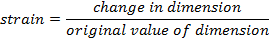S = strain (unitless)

Δx = change in dimension (m for longitudinal or shearing strain, m3 for volumetric strain)

X = original dimension (m for longitudinal or shearing strain, m3 for volumetric strain)

Strain Formula (general form) Questions:

1) Heating causes metals to expand. A hot liquid is put through a copper pipe 10.00 m long. This causes the length to increase to 10.17 m. What is the longitudinal strain?

Answer: The longitudinal strain is the change in length divided by the original length. The change in length is the difference between the final length (l2) and the initial length (l1). The strain can be found using the formula: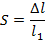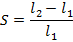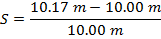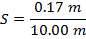S = 0.017

The longitudinal strain is 0.017.

2) As a certain quantity of gas is subjected to high pressure, its volume decreases from 40.0 m3 to 2.0 m3. What is the volumetric strain?

Answer: The volumetric strain is the change in volume divided by the original volume. The change in volume is the difference between the final volume (V2) and the initial volume (V1). The strain can be found using the formula: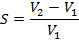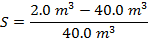S = -0.950

The volumetric strain is -0.950.